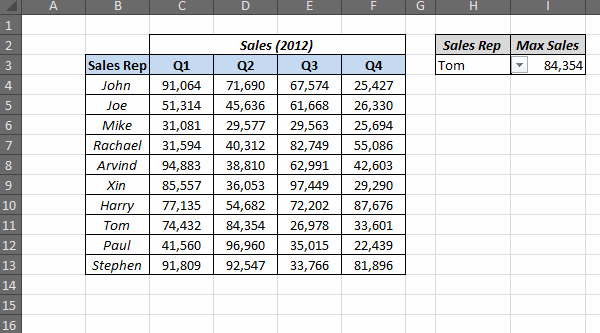# Excel: Lookup and Return Values in an Entire Row/Column

VLOOKUP is one of the most used functions in Excel. It looks for a value in a range and returns a corresponding value in a specified column number.
Now I came across a problem where I had to lookup entire row and return the values in all the columns from that row (instead of returning a single value).
So here is what I had to do. In the below data set, I had Sales Rep names and the Sales they made in 4 quarters in 2012. I had a drop down with their names, and I wanted to extract the maximum sales for that Sales Rep in those four quarters.
xI could come up with 2 different ways to do this – Using INDEX or VLOOKUP.

#### Lookup Entire Row / Column Using INDEX Formula

Here is the formula I created to do this using Index
`=LARGE(INDEX(\$B\$4:\$F\$13,MATCH(H3,\$B\$4:\$B\$13,0),0),1)`
##### How it works:
Let first look at the INDEX function that is wrapped inside the LARGE function.
=INDEX(\$C\$4:\$F\$13,MATCH(H3,\$B\$4:\$B\$13,0),0)
Let's closely analyze the arguments of the INDEX function:
• Array – \$B\$4:\$F\$1
• Row Number –  MATCH(H3,\$B\$4:\$B\$13,0)
• Column Number – 0
Notice that I have used column number as 0.
The trick here is that when you use column number as 0, it returns all the values in all the columns. So if I select John in the drop down, the index formula would return all the 4 sales values for John {91064,71690,67574,25427}.
Now I can use the Large function to extract the largest value
ExcelTip #1 – Use Column/Row number as 0 in Index formula to return all the values in Columns/Rows.#### Lookup Entire Row / Column Using VLOOKUP Formula

While Index formula is neat, clean and robust, VLOOKUP way is a bit complex. It also ends up making the function volatile. However, there is an amazing trick that I would share in this section. Here is the formula:
`=LARGE(VLOOKUP(H3,B4:F13, ROW(INDIRECT("2:"&COUNTA(\$B\$4:\$F\$4))), FALSE),1) `
##### How it works
• ROW(INDIRECT("2:"&COUNTA(\$B\$4:\$F\$4))) – This formula returns an array {2;3;4;5}. Note that since it uses INDIRECT, this makes this formula volatile.
• VLOOKUP(H3,B4:F13,ROW(INDIRECT("2:"&COUNTA(\$B\$4:\$F\$4))),FALSE) – Here is the best part. When you put these together, it becomes VLOOKUP(H3,B4:F13,{2;3;4;5},FALSE). Now notice that instead of a single column number, I have given it an array of column numbers. And VLOOKUP obediently looks up values in all these columns and returns an array.
• Now just use LARGE function to extract the largest value.
Remember to use Control + Shift + Enter to use this formula.
ExcelTip #2 – In VLOOKUP, instead of using a single column number, if you use an array of column numbers, it will return an array of lookup values.
###### Related Tutorials: